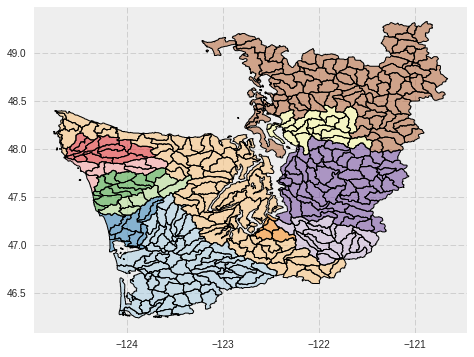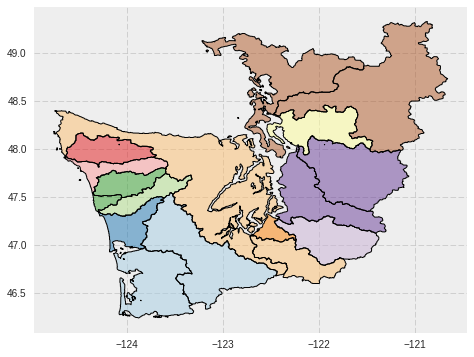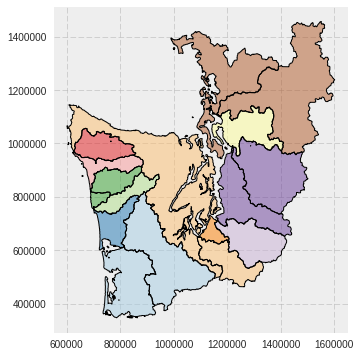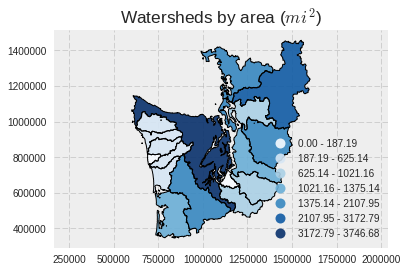Emilio Mayorga, 2017-2-6

## 1. Introduction¶

We covered the basics of GeoPandas in the previous episode and notebook. Here, we'll extend that introduction to illustrate additional aspects of GeoPandas and its interactions with other Python libraries, covering fancier mapping, analysis (unitary and binary spatial operators), raster zonal stats and geopandas.

Here are the main sections in this episode / notebook:

• Read HydroBASINS Pacific NW / Western Washington from a shape file.
• Dissolve into larger watersheds, and reproject
• Plot choropleth map based on calculated watershed areas
• Choropleth map as an interactive map with folium
• Spatial join, sjoin, of polygons on points
• rasterstats: "zonal" statistics from polygons on rasters

## 2. Set up packages and data file path¶

We'll use these throughout the rest of the tutorial.

In :
%matplotlib inline

import os
import json

import matplotlib.pyplot as plt
# The two statemens below are used mainly to set up a plotting
# default style that's better than the default from matplotlib
import seaborn as sns
plt.style.use('bmh')

from shapely.geometry import Point
import pandas as pd
import geopandas as gpd
from geopandas import GeoSeries, GeoDataFrame

data_pth = "../data"


## 3. Read HydroBASINS North America / Western Washington¶

• Read HydroBASINS "all-levels" (lev00) hierarchical watersheds dataset for the Pacific NW, from a shape file. Watersheds in the dataset are at the finest (highest resolution) "Pfastetter" hierarchical level, level 12.
• In the original UW GeoHack Week tutorial, this exercise involved reading geospatial data from a remote PostGIS source.
In :
hydrobas = gpd.read_file(os.path.join(data_pth, "hybas_na_lev00_v1c.shp"))

In :
len(hydrobas)

Out:
14866

We'll apply a filter to the dataset to select only the Pfastetter level-4 watershed with code 7831: hydrobas['pfaf_4'] == 7831. This is most of Western Washington. Watershed polygons will still be read at their original level 12 resolution.

In :
hydrobas_ww = hydrobas[hydrobas['pfaf_4'] == 7831]


413 polygon features returned. Let's examine the attributes for the first feature.

In :
len(hydrobas_ww)

Out:
413
In :
hydrobas_ww.iloc

Out:
coast                                                        0
dist_main                                                    0
dist_sink                                                    0
endo                                                         0
geometry     POLYGON ((-123.35 46.36666666666669, -123.3493...
hybas_id                                            2147483647
main_bas                                            2147483647
next_down                                                    0
next_sink                                           2147483647
order                                                        1
pfaf_1                                                       7
pfaf_10                                             2147483647
pfaf_11                                             2147483647
pfaf_12                                             2147483647
pfaf_2                                                      78
pfaf_3                                                     783
pfaf_4                                                    7831
pfaf_5                                                   78310
pfaf_6                                                  783101
pfaf_7                                                 7831010
pfaf_8                                                78310101
pfaf_9                                               783101010
sort                                                     19945
sub_area                                                 135.4
up_area                                                  135.4
Name: 3908, dtype: object

Plot a categorical map, with coloring based on the aggregating column pfaf_7. Watershed boundaries are at the high-resolution Pfastetter level 12.

In :
hydrobas_ww.plot(column='pfaf_7', cmap='Paired', categorical=True, figsize=(14,6));## 4. Dissolve into larger watersheds, and reproject¶

### Dissolve source polygons into larger watersheds based on attribute values¶

Apply GeoDataFrame dissolve aggregation method (implemented from lower level shapely operators) on level-7 Pfastetter codes (pfaf_7) shown in the plot above. Aggregate attributes, retaining only pfaf_7 and pfaf_6 (plus geometry, of course). Note that this operation results in only 17 polygons, from the original 413.

In :
cols = ['pfaf_6', 'pfaf_7', 'geometry']
hydrobas_ww_p7 = hydrobas_ww[cols].dissolve(by='pfaf_7', aggfunc='first', as_index=False)
len(hydrobas_ww_p7)

Out:
17
In :
hydrobas_ww_p7.crs


The CRS was not retained during the dissolve operation. Apply it by copying it from hydrobas_ww.

In :
hydrobas_ww_p7.crs = hydrobas_ww.crs
hydrobas_ww_p7.crs

Out:
{'init': u'epsg:4326'}

Let's examine some of the features.

In :
hydrobas_ww_p7.head()

Out:
pfaf_7 geometry pfaf_6
0 7831010 (POLYGON ((-123.5722222222222 46.2458333333333... 783101
1 7831020 POLYGON ((-123.1791666666666 46.33333333333336... 783102
2 7831031 (POLYGON ((-123.9597222222222 46.9666666666667... 783103
3 7831032 POLYGON ((-123.8583333333333 47.39583333333336... 783103
4 7831033 POLYGON ((-124.3 47.34583333333336, -124.30221... 783103

Plot the results. Looks like the previous plot, except the polygon boundaries are now the pfaf_7 watersheds (same as the background colors).

In :
hydrobas_ww_p7.plot(column='pfaf_7', cmap='Paired', categorical=True, figsize=(14,6));NOTE/WATCH:
Beware that dissolve may fail if there are "invalid" geometries. See this code from the previous, intro notebook. The 6 geometries/points reported are invalid (and are reported by the is_valid() method). This dissolve statement does work, though.

seas_grp = seas[['oceans', 'geometry']]
seas_oceans_diss = seas_grp[seas_grp.geometry.is_valid].dissolve(by='oceans')

Ring Self-intersection at or near point 10.407218181818182 54.821390909090908
Self-intersection at or near point -79.365827272727287 76.296645454545455
Ring Self-intersection at or near point 10.979445510225332 54.380555030408686
Ring Self-intersection at or near point 133.61550925464189 -4.3005540903175188
Ring Self-intersection at or near point 121.91067196634913 -5.0593090510592447
Ring Self-intersection at or near point 115.29553592754269 -7.0082630551828515


### Reproject (transform) to WA State Plane South, epsg:2927¶

Partly so we can calculate polygon areas in linear units, not geodetic degrees. But also because that's the projection used by most state and local governments in Washington.

No need to go to a web site to learn more about what epsg:2927 is. Use pyepsg, which issues queries to http://epsg.io web services.

In :
import pyepsg


Extract the epsg code from the string returned by crs['init'].

In :
hydrobas_ww_p7_epsg_str = hydrobas_ww_p7.crs['init'].split(':')
pyepsg.get(hydrobas_ww_p7_epsg_str)

Out:
<GeodeticCRS: 4326, WGS 84>

Query epsg 2927.

In :
pyepsg.get('2927')

Out:
<ProjectedCRS: 2927, NAD83(HARN) / Washington South (ftUS)>

Apply the crs transformation (reprojection) using to_crs method.

In :
hydrobas_ww_p7_wasp = hydrobas_ww_p7.to_crs(epsg=2927)


#### Plot the reprojected map.¶

Note that, being in a planar project (not geodetic), the shape looks different compared to the previous map. More "normal". And the axes are now in feet relative to some origin.

In :
hydrobas_ww_p7_wasp.plot(column='pfaf_7', cmap='Paired', categorical=True, figsize=(14,6));## 5. Plot choropleth map based on calculated watershed areas¶

As the projection is in feet, auto-calculated polygon areas will be in feet2. So let's convert to miles2 first (why not!). We'll add a new column to the GeoDataFrame.

In :
hydrobas_ww_p7_wasp['area_mi2'] = hydrobas_ww_p7_wasp.geometry.area / 27878400

Out:
pfaf_7 geometry pfaf_6 area_mi2
0 7831010 (POLYGON ((863343.6925921033 347890.2242189167... 783101 1375.137396
1 7831020 POLYGON ((963803.8027083561 376154.9965688475,... 783102 2107.945774
2 7831031 (POLYGON ((776917.1877152125 614568.383332147,... 783103 528.472846

NOTE/FUN:
Now you could get the area of a pfaf_6 watershed via simple Pandas DataFrame groupby aggregation (sum).

Plot the choloropleth, using area_mi2.

"fisher_jenks" value segmentation scheme (using 7 segments, k=7) used is one of the available pysal.esda.mapclassify.Map_Classifier classifiers from the powerful PySAL package (Python Spatial Analysis Library); GeoPandas can use these classifiers if PySAL is installed. To get the list of classifiers, use:

import pysal
print(pysal.esda.mapclassify.Map_Classifier.__doc__)

In :
f, ax = plt.subplots(1, figsize=(6, 4))
hydrobas_ww_p7_wasp.plot(column='area_mi2', scheme='fisher_jenks', k=7,
alpha=0.9, cmap=plt.cm.Blues, legend=True, ax=ax)
plt.axis('equal')
ax.set_title('Watersheds by area ($mi^2$)');## 6. Choropleth map as an interactive map with folium¶

Folium is very cool, specially for use in Jupyter notebooks; or to export into stand-alone HTML.

In :
import folium


Folium likes GeoJSON for geometries. GeoPandas makes conversion easy.

In :
geo_str = hydrobas_ww_p7.to_json()


m.choropleth will do an attribute join between geo_str and hydrobas_ww_p7_wasp based on the key pfaf_7.

In :
m = folium.Map(location=[47.8, -122.5], zoom_start=7,
tiles="cartodbpositron")

m.choropleth(geo_str=geo_str,
data=hydrobas_ww_p7_wasp, columns=['pfaf_7', 'area_mi2'],
key_on='feature.properties.pfaf_7',
fill_color='YlGn', fill_opacity=0.4,
legend_name='area')
m

/home/mayorga/miniconda/envs/geopandasenv/lib/python2.7/site-packages/ipykernel/__main__.py:8: FutureWarning: 'threshold_scale' default behavior has changed. Now you get a linear scale between the 'min' and the 'max' of your data. To get former behavior, use folium.utilities.split_six.

Out: